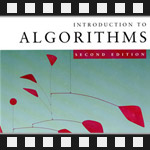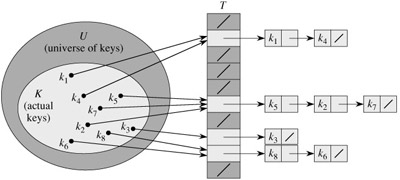This is the fifth post in an article series about MIT's lecture course "Introduction to Algorithms." In this post I will review lectures seven and eight, which are on the topic of Hashing.

Many applications require a dynamic set that supports dictionary operations insert, search, and delete. For example, a compiler for a computer language maintains a symbol table, in which the keys of elements are arbitrary strings that correspond to identifiers in the language. A hash table is an effective data structure for implementing dictionaries.

Lectures seven and eight cover various implementation techniques of hash tables and hash functions.

## Lecture 7: Hashing I

Lecture seven starts with the symbol-table problem -- given a table S, we'd like to insert an element into S, delete an element from S and search for an element in S. We'd also like these operations to take constant time.

The simplest solution to this problem is to use a direct-access (or direct-address) table. To represent the dynamic set, we use an array, or direct-address table, denoted by T, in which each position, or slot, corresponds to a key.

Using direct-address table, the dictionary operations are trivial to implement.

```Direct-Address-Search(T, k)
return T[k]

T[key[x]] = x

T[key[x]] = NIL
```

Direct addressing is applicable when we can afford to allocate an array that has one position for every possible key. It is not applicable when the range of keys can be large (as it requires a lot of space for the array T). This is where hashing comes in.

The lecture continues with explanation of what hashing is. Hashing uses a hash function h(k) that maps keys k randomly into slots of hash-table T. There is one hitch: two keys may hash to the same slot. We call this situation a collision. Fortunately, there are effective techniques for resolving the conflict created by collisions.

One of the simplest collision resolution techniques is called chaining. In chaining, we put all the elements that hash to the same slot in a linked list:Collision resolution by chaining. Each hash-table slot T[j] contains a linked list of all the keys whose hash value is j. For example, h(k1) = h(k4).

Professor Leiserson then analyzes the running time of insert, delete and search operations. It is concluded that the expected running time operations is still O(1), under assumption that the number of hash-table slots is at least proportional to the number of elements in the table.

The other half of the lecture is devoted to hash functions and another way of resolving collisions -- resolving collisions by open addressing, and probing strategies (search) for open addressing -- linear probing and double hashing.

A good hash function should distribute the keys uniformly into the slots of the table and the regularity in the key distributions should not affect uniformity.

Two hash function creating methods are introduced - the division method, which defines h(k) = k mod m, and the multiplication method, where h(k) = (A·k mod 2w)>>(w-r), where w is bits in a word, A is an odd integer between 2w-1 and 2w, and r is lg(m).

You're welcome to watch lecture seven:

Topics covered in lecture seven:

• [00:30] Symbol-table problem.
• [02:05] Symbol-table operations: insert, delete, search.
• [09:45] Hashing.
• [14:30] Resolving hash function collisions by chaining.
• [17:05] Worst-case analysis of chaining.
• [19:15] Average-case analysis of chaning.
• [29:30] Choosing a hash function.
• [30:55] Division method hash function.
• [39:05] Multiplication method hash function.
• [46:30] Multiplication method explained with a modular wheel.
• [50:12] Resolving hash function collisions by open addressing.
• [59:00] Linear probing strategy.
• [01:01:30] Double hashing probing strategy.
• [01:04:20] Average-case analysis of open addressing.

Lecture seven notes:

## Lecture 8: Hashing II

Lecture eight starts with addressing a weakness of hashing -- for any choice of hash function, there exists a set of keys that all hash to the same value. This weakness can lead to denial of service attacks on the application using hashing.

The idea of addressing this problem is to choose a hash function at random! This is called universal hashing.

The lecture then moves to a mathematically rigorous the definition of universal hashing and explains one of many ways to construct a universal hash function.

The other half of the lecture is devoted to perfect hashing. Perfect hashing solves a problem of constructing a static hash table (such as a hash table stored on a CD), so that searches take O(1) time guaranteed (worst-case). The key idea in creating such hash table is to use 2-level universal hashing, so that no collisions occur in level 2.

Video of lecture eight:

Topics covered in lecture eight:

• [00:30] Fundamental weakness of hashing.
• [05:12] Universal hashing.
• [20:10] Constructing a universal hash function.
• [49:45] Perfect hashing.
• [54:30] Example of perfect hashing.
• [01:06:27] (Markov inequality)
• [01:14:30] Analysis of storage requirements for perfect hashing.

Lecture eight notes:

Have fun hashing! The next post will be about random binary search trees and red-black trees.